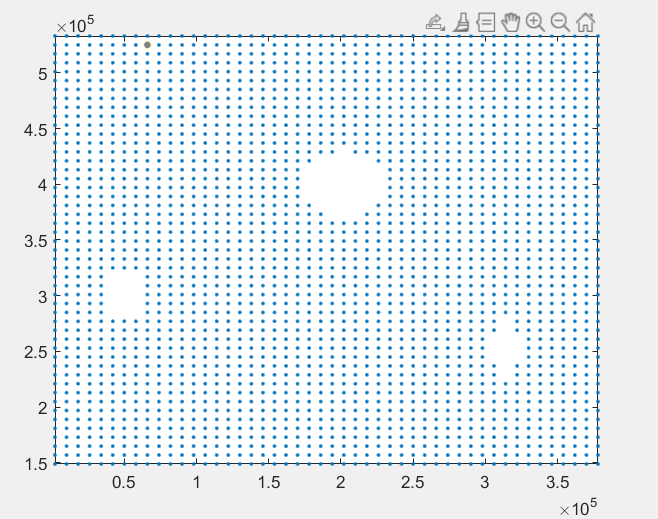# fill missing point in data (x y z 3 columns data)

3 views (last 30 days)
h k on 13 Sep 2021
Commented: Rena Berman on 20 Sep 2021
hello.
I have a data with three columns that have empty spaces inside.
For example, a data has 2500 rows (point) and its length and width are 50 x 60 = 3000
I want to grid this data and put (nan) in place of the blanks
The following plot is drawn with the plot(x,y,'.')Rena Berman on 20 Sep 2021

Dave B on 14 Sep 2021
You can use meshgrid to make x and y grids, and then ismember with the rows flag to find which pairs of x and y are missing:
% Some fake data that simulares your problem
[x,y]=meshgrid(1:10,1:10);
data=[x(:) y(:) rand(numel(x),1)];
% Remove 10 random indices
data(randperm(numel(x),10),:)=[];
scatter(data(:,1),data(:,2),'filled')
% Make a grid using meshgrid, you'll need at least one point for each grid
% location in either x or y. Otherwise, specify the grid x and y
% explicitly for the first two arguments of meshgrid.
[xi,yi]=meshgrid(unique(data(:,1)), unique(data(:,2)));
notfound = ~ismember([xi(:) yi(:)],data(:,1:2),'rows');
data=[data; xi(notfound) yi(notfound) nan(sum(notfound),1)];
hold on
scatter(data(:,1),data(:,2),75)
legend('Pre','Post','Location','EastOutside')Chunru on 14 Sep 2021
Edited: Chunru on 14 Sep 2021
% Generate some data
[x, y] = meshgrid(1:60, 1:50);
x = x(:);
y = y(:);
z = 1./((x-30).^2+(y-20).^2);
% make a square hole
[ix, iy] = meshgrid(20:30, 30:45);
ix = ix(:); iy=iy(:);
idx = sub2ind([50, 60], iy, ix);
x(idx)=[]; y(idx)=[]; z(idx)=[];
% Replace x,y,z with your data
plot(x, y, '*');% get the grid from data x and y
xg = sort(unique(x));
yg = sort(unique(y));
[xg, yg] = meshgrid(xg, yg);
idx = ~ismember([xg(:) yg(:)], [x y], 'rows');
%idx(:)
xg(idx) = nan;
yg(idx) = nan;
figure
imagesc(isnan(xg) & isnan(yg))
axis xy% Assign z
zg = nan(size(xg));
zg(~idx) = z;
figure
%imagesc(zg);
%axis xy
surfl(zg)figure
imagesc(isnan(zg))
axis xywhos
Name Size Bytes Class Attributes idx 3000x1 3000 logical ix 176x1 1408 double iy 176x1 1408 double x 2824x1 22592 double xg 50x60 24000 double y 2824x1 22592 double yg 50x60 24000 double z 2824x1 22592 double zg 50x60 24000 double